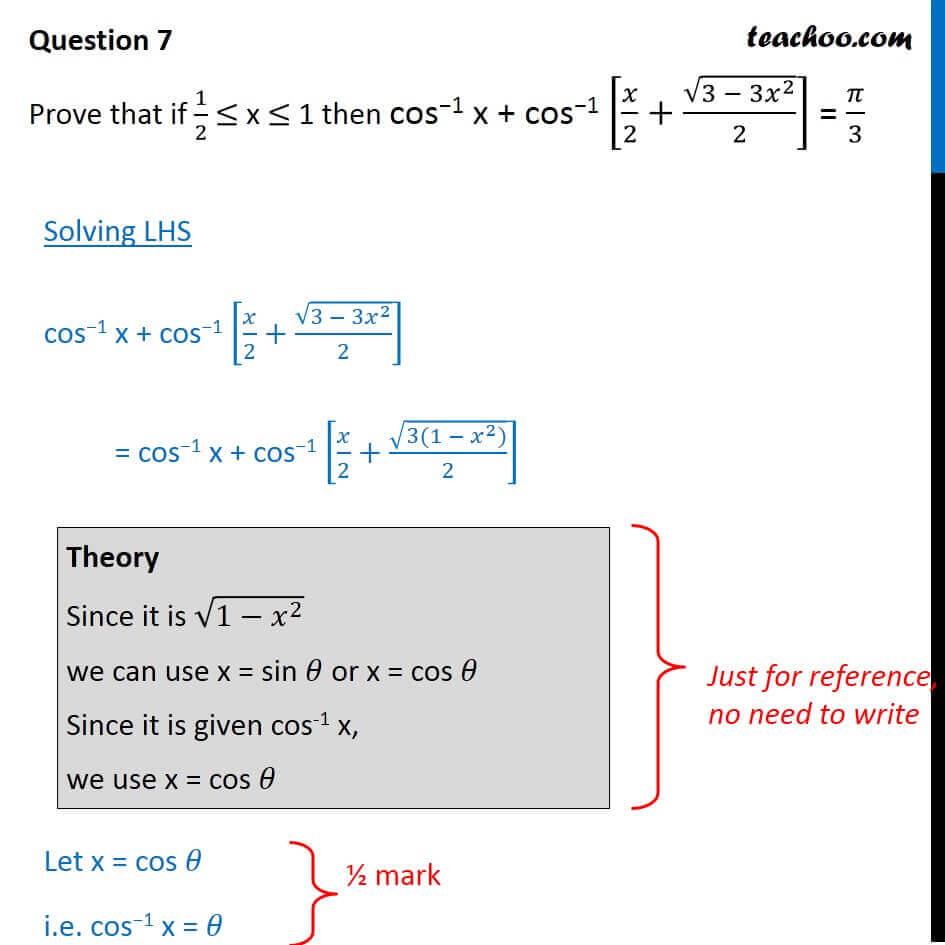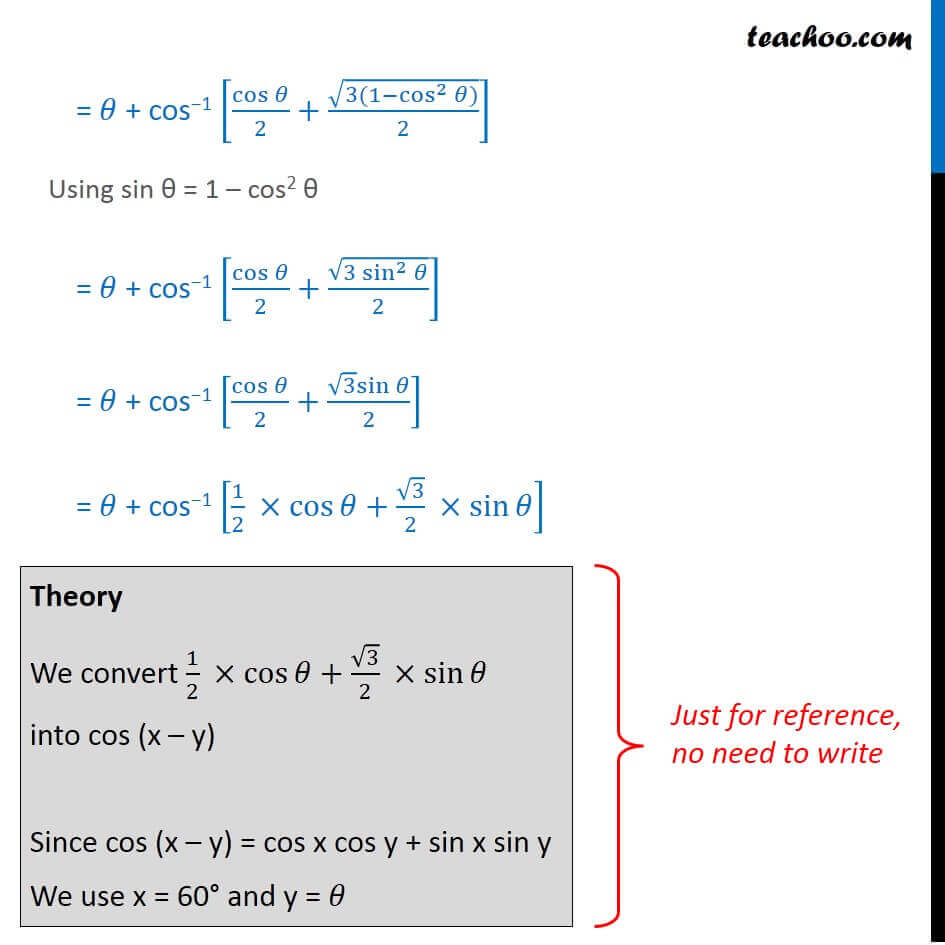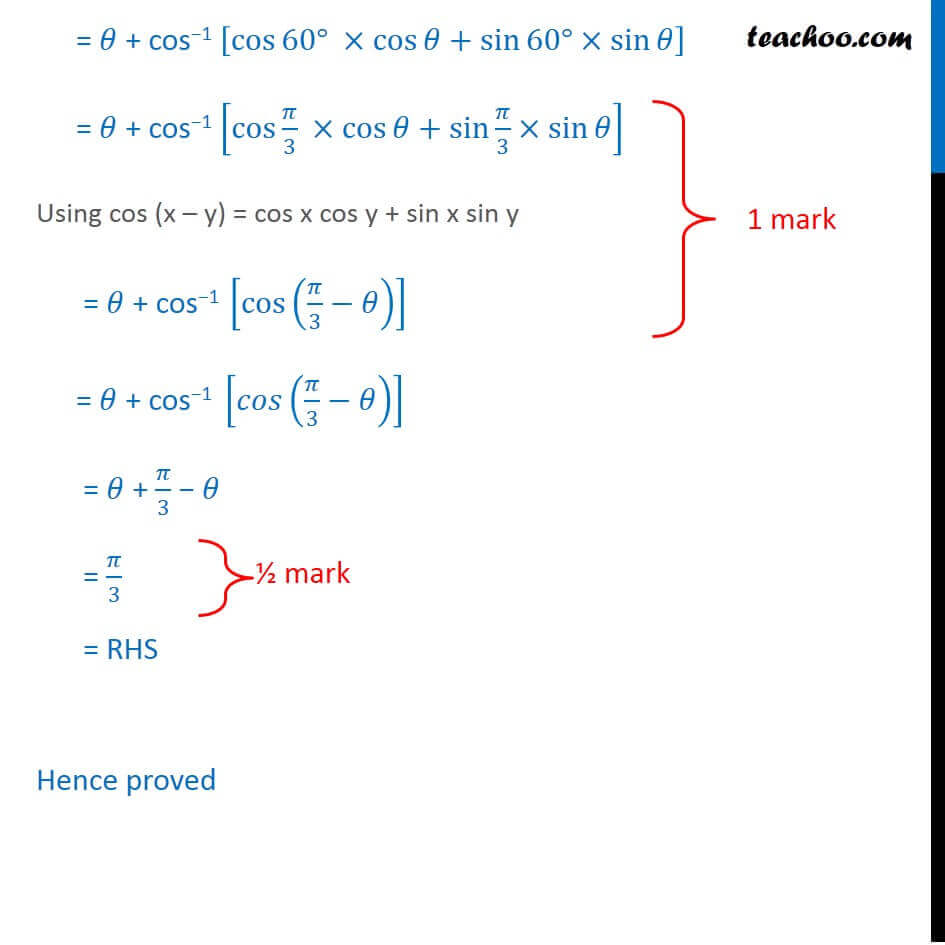CBSE Class 12 Sample Paper for 2018 Boards

Class 12
Solutions of Sample Papers and Past Year Papers - for Class 12 Boards

### Prove that if 1/2 ≤ x ≤ 1, then cos −1 x + cos −1 [x/2+√(3 - 3x 2 )/2] = π/3

This is a question of CBSE Sample Paper - Class 12 - 2017/18.Learn in your speed, with individual attention - Teachoo Maths 1-on-1 Class

### Transcript

Question 7 Prove that if 1/2 ≤ x ≤ 1 then cos−1 x + cos−1 [𝑥/2+√(3 − 3𝑥^2 )/2] = 𝜋/3 Solving LHS cos−1 x + cos−1 [𝑥/2+√(3 − 3𝑥^2 )/2] = cos−1 x + cos−1 [𝑥/2+√(3(1 − 𝑥^2))/2] Let x = cos 𝜃 i.e. cos−1 x = 𝜃 Theory Since it is √(1−𝑥^2 ) we can use x = sin 𝜃 or x = cos 𝜃 Since it is given cos-1 x, we use x = cos 𝜃 = 𝜃 + cos−1 [cos⁡𝜃/2+√(3(1−cos^2⁡〖𝜃)〗 )/2] Using sin θ = 1 – cos2 θ = 𝜃 + cos−1 [cos⁡𝜃/2+√(3 sin^2⁡𝜃 )/2] = 𝜃 + cos−1 [cos⁡𝜃/2+〖√3 sin〗⁡𝜃/2] = 𝜃 + cos−1 [1/2 ×cos⁡𝜃+√3/2 ×sin⁡𝜃 ] Theory We convert 1/2 ×cos⁡𝜃+√3/2 ×sin⁡𝜃 into cos (x – y) Since cos (x – y) = cos x cos y + sin x sin y We use x = 60° and y = 𝜃 = 𝜃 + cos−1 [cos⁡〖60°〗 ×cos⁡𝜃+sin⁡〖60°〗×sin⁡𝜃 ] = 𝜃 + cos−1 [cos⁡〖𝜋/3〗 ×cos⁡𝜃+sin⁡〖𝜋/3〗×sin⁡𝜃 ] Using cos (x – y) = cos x cos y + sin x sin y = 𝜃 + cos−1 [cos⁡(𝜋/3−𝜃) ] = 𝜃 + cos−1 [𝑐𝑜𝑠(𝜋/3−𝜃)] = 𝜃 + 𝜋/3 − 𝜃 = 𝜋/3 = RHS Hence proved